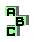NINECENTER   (1/6)

 r5 first Brocard triangle r37 Napoleon points, isodynamic points, orthocenter, circumcenter r38 Napoleon points, circumcenter, Fermat points r40 Vecten points, symmedian point d Lester circle d Kosnita point r133 Fermat points, Napoleon points, circumcenter, symmedian point, Kiepert hyperbola, Evans conic, autopolar triangle r140 point X(12), incenter, Feuerbach point, harmonic points r146 Tarry point, third Brocard point, symmedian point, point X(262) r188 incenter, Spieker point, circumcenter, Fuhrmann point r192 altimedial triangles, incircle, radical center r209 orthocentroidal circle, circumcenter, inverse points r218 Nagel point, De Longchamps point, orthocenter, Euler line, Soddy line r254 Simson line, circumtangential triangle r308 point X(110), point X(125), point X(265), circumcenter r324 point X(265), Euler line, cevian product r337 Prasolov point, orthic triangle, perspective r366 Feuerbach point, point X(12), desmic structure r373 Feuerbach point, perspective, anticevian triangle, cevian quotient r374 point X(12), perspective,anticevian triangle, cevian quotient r472 incenter, orthocenter, circumcenter r473 incenter, Fuhrmann triangle, homothetic triangles1 2 3 4 5 6